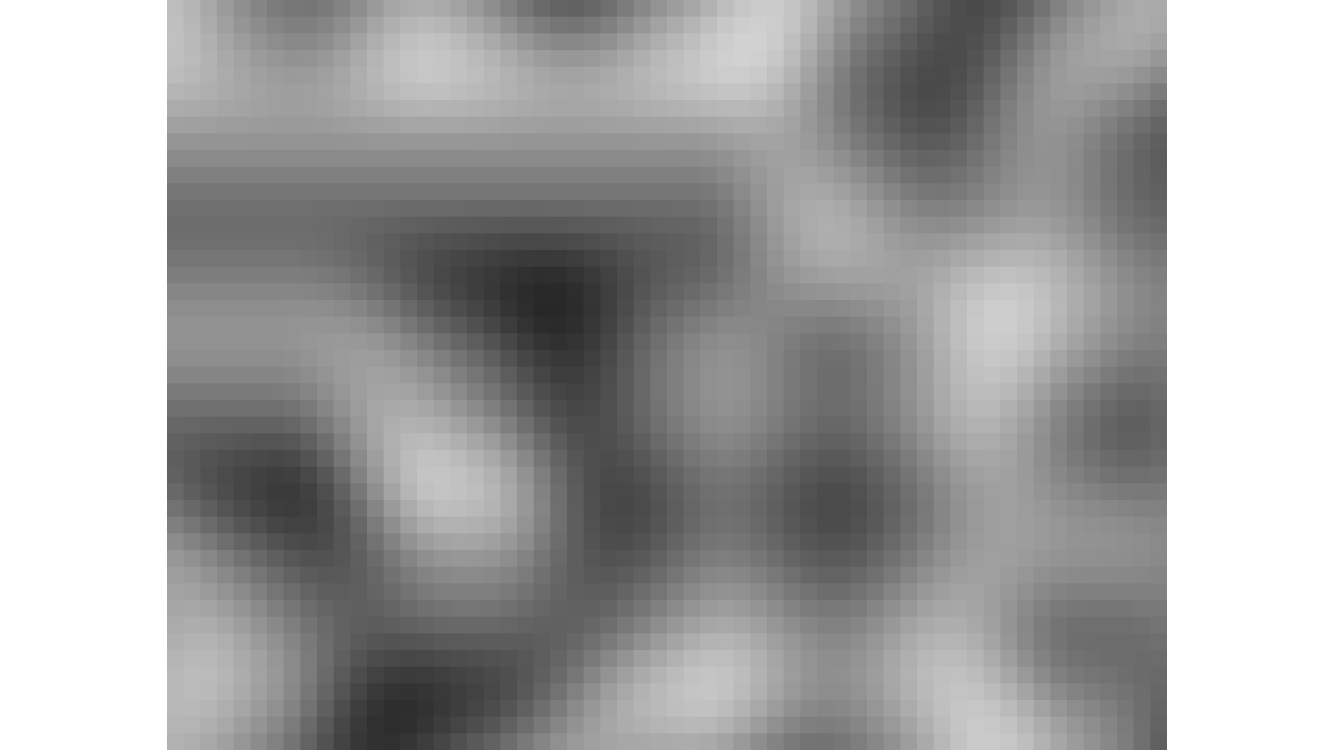# Swiftpack.co - hirota1ro/swift-noise-generator as Swift Package

Swiftpack.co is a collection of thousands of indexed Swift packages. Search packages.
hirota1ro/swift-noise-generator 1.0.0
This library project contains a few noise generators created in Swift.
⭐️ 0
🕓 2 weeks ago
.package(url: "https://github.com/hirota1ro/swift-noise-generator.git", from: "1.0.0")

# SwiftNoiseGenerator

This library project contains a few noise generators written in Swift.

contains:

• Perlin Noise
• Simplex Noise

screenshot:## How to use

### To use from an executable package

Edit `Package.swift` to define the dependencies.

``````     name: "MyExecutable",
dependencies: [
// Dependencies declare other packages that this package depends on.
-        // .package(url: /* package url */, from: "1.0.0"),
+        .package(url: "https://github.com/hirota1ro/swift-noise-generator", from: "1.0.0"),
],
targets: [
// Targets are the basic building blocks of a package. A target can define a module or a test suite.
// Targets can depend on other targets in this package, and on products in packages this package depends on.
.executableTarget(
name: "MyExecutable",
-            dependencies: []),
+            dependencies: [.product(name: "SwiftNoiseGenerator", package: "swift-noise-generator")]),
.testTarget(
name: "MyExecutableTests",
dependencies: ["MyExecutable"]),
``````

for example `main.swift`

``````import Foundation
import SwiftNoiseGenerator

let generator = PerlinNoise() // or SimplexNoise()

var a: [String] = []
for j in stride(from: 0, to: 360, by: 8) {
for i in stride(from: 0, to: 480, by: 8) {
let x = Double(i - 240) / 100
let y = Double(j - 180) / 100

let noise = generator.normalized(x, y, 0) // 0〜1
let b = Int(noise * 255) // 0x00〜0xFF
let rgb = (b << 16) | (b << 8) | (b) // 0x000000〜0xFFFFFF
let hex = String(format: "%06x", rgb)

let s = """
<rect x="\(i)" y="\(j)" width="8" height="8" fill="#\(hex)" stroke="none" />
"""
a.append(s)
}
}

let svg = """
<svg width="480" height="360" viewBox="0 0 480 360" xmlns="http://www.w3.org/2000/svg" version="1.1">
\(a.joined(separator: "\n"))
</svg>
"""
let file = dir.appendingPathComponent("noise.svg")
try! svg.write(to: file, atomically: true, encoding: .utf8)
``````

### To use from an Xcode project

Xcode → File → Add Packages → input this repository URL.

Here is a sample code.

``````import UIKit
import SwiftNoiseGenerator

...

func createImage() -> UIImage {
return UIGraphicsImageRenderer(size: CGSize(width: 480, height: 360)).image { _ in
let generator = PerlinNoise()
for j in stride(from: 0, to: 360, by: 8) {
for i in stride(from: 0, to: 480, by: 8) {
let x = CGFloat(i - 240) / 100
let y = CGFloat(j - 180) / 100
let brightness = generator.normalized(x, y, 0)
let color = UIColor(white: brightness, alpha: 1)
color.setFill()
let path = UIBezierPath(rect: CGRect(x: CGFloat(i), y: CGFloat(j), width: 8, height: 8))
path.fill()
}
}
}
}
``````

### GitHub

 link Stars: 0 Last commit: 1 week agoSomething's broken? Yell at me @ptrpavlik. Praise and feedback (and money) is also welcome.

Swiftpack is being maintained by Petr Pavlik | @ptrpavlik | @swiftpackco | API | Analytics